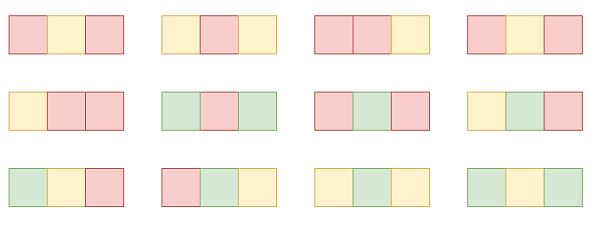# Number of Ways to Paint N × 3 Grid in C++

Suppose we have grid of size n x 3 and we want to paint each cell of the grid with exactly one of the three colors. The colors are Red, Yellow or Green. Now there is a constraint that is no two adjacent cells have the same color. We have n the number of rows of the grid. We have to find the number of ways we can paint this grid. The answer may be very large so return it modulo 10^9 + 7.

So, if the input is like 1, then the output will be 12To solve this, we will follow these steps −

• m = 1^9 + 7

• Define a function add(), this will take a, b,

• return ((a mod m) + (b mod m)) mod m

• From the main method do the following −

• a123 := 6, a121 = 6

• for initialize i := 2, when i <= n, update (increase i by 1), do −

• b121 := add(3 * a121, 2 * a123)

• b123 := add(2 * a121, 2 * a123)

• a121 := b121

• a123 := b123

Let us see the following implementation to get better understanding −

## Example

Live Demo

#include <bits/stdc++.h>
using namespace std;
typedef long long int lli;
const lli mod = 1e9 + 7;
class Solution {
public:
return ((a % mod) + (b % mod)) % mod;
}
int numOfWays(int n){
lli a123 = 6, a121 = 6;
lli b123, b121;
for (int i = 2; i <= n; i++) {
b121 = add(3 * a121, 2 * a123);
b123 = add(2 * a121, 2 * a123);
a121 = b121;
a123 = b123;
}
}
};
main(){
Solution ob;
cout << (ob.numOfWays(3));
}

## Input

3

## Output

246